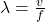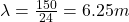## One end of a nylon rope is tied to a brick wall. The rope is stretched horizontally, passed over a pulley 80 meters away, and weighted with

Question

One end of a nylon rope is tied to a brick wall. The rope is stretched horizontally, passed over a pulley 80 meters away, and weighted with a mass 180.0 kg at the end. Waves on the rope are measured, and found to travel at 150 m/s. What is the mass of the rope between wall and pulley? If the rope is shaken sideways at 24.0 Hz, what is the wavelength of the waves produced?

in progress 0
7 months 2021-07-20T20:49:06+00:00 1 Answers 5 views 0

Mass of the rope m = 6.272 kg

Wavelength is equal to 6.25 m

Explanation:

Length of pulley L = 180 M

Mass at the end of pulley m = 180 kg

Frequency is given f = 24 Hz

Acceleration due to gravity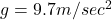Tension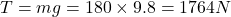Velocity of wave in the rope v = 150 m/sec

Velocity of wave is given by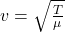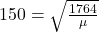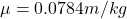Therefore mass of the rope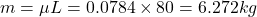Wavelength is equal to